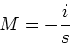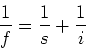PHY294H - Lecture 40

Vision

Before we start analysing mirrors, it is useful to first understand a little bit about how human eyes and the human brain provides us with depth perception. To understand this, and many other issues in geometrical optics, we need to first understand how we perceive the distance to a point source. The light from a point source reaches the retina of our two eyes at different positions. The brain notices this disparity'' and uses it to estimate the distance to the source. To a lesser extent this can works for one eye however the resolution is much worse. Even the resolution of distance to a point source using the disparity in two eyes does not give high resolution. Our eyes provide excellent depth resolution when the landscape has many features. The brain uses all of these features as landmarks in forming an overall perception of the scene. Depth resolution is much worse when those features are absent, for example a point source of light on a dark night. Disparity is due to diverging rays of light. By tracing these rays back to their source we perceive depth. Subconsciously our brain carries out this process all the time. Now we have to do it consciously. The focus of our analysis is thus to find points from which light appears to emanate. These points are perceived by our brains as an object''. If they are not a true object or source of the original light, we call them an image.

The bottom line: If light rays diverge from a common point, our eyes locate the image at that common source''.

All of our analysis in geometrical optics thus focuses on identifying points at which light rays converge and then diverge. These points are identified as images. We will be interested in controlling the location and size of these images.

Two main results for plane mirrors

1. If a point object is placed a distance d in front of a plane mirror, it produces a virtual image a distance d behind the mirror.

2. The image is reversed.

Note that our eyes cannot distinguish between the virtual image and the real object (with no mirror!) at that same location. Thus if we now place a second mirror in front of the first, the rays from the virtual image are reflected as if the virtual image were a real object. It is thus evident that we can construct ray diagrams recursively, treating one mirror at a time. This is very helpful in analysing complex geometrical systems.

Spherical mirrors

Concave mirror : A section of the interior surface of a sphere.

Principle rays used to identify images in mirrors

1. A ray parallel to the axis reflects through the focal point.

2. A ray through the center of curvature of the spherical mirror reflects directly back to the point of origin.

3. A ray reflecting from the point where the central axis meets the mirror reflects symmetrically.

4. A ray through the focal point reflects parallel to the central axis.

From the ray construction of the image, it is possible to find the key relations,(1)

and(2)

where:

- f is the focal length

- s is the object distance

- i is the image distance

- M is the magnification

Sign conventions for mirrors

1. A real image is on the same side of the mirror as the source. A virtual image is behind the mirror.

2. Real images have positive image distance, virtual images have negative image distance.

3. Inverted images have negative magnification. Upright images have positive magnification.

4. Diverging (convex) mirrors have negative focal length.

Convex mirror: The exterior of a section of a sphere.

Summary of results for spherical mirrors

You need to know have to use ray diagrams to illustrate each of these cases.

Concave mirrors

- If s>R, the image is real, inverted and reduced in size.

- If f<s<R, the image is real inverted and magnified.

- If s<f, the image is virtual, upright and magnified.

Convex mirrors

- For all object(source) distances, the image is virtual, upright and reduced in size.